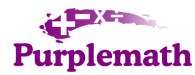Search

Homework Guidelines: Examples

1) Determine whether x = 0 is a solution of 5x  3 = 3x + 5.

[unacceptable solution]

N                                 Hello?  "N"?  What does that mean?

[acceptable solution]

 5x  3 = 3x + 5 Ah, much better! Now we can see how todo this problem. Also, we can clearly seehow to interpret thecomputational result,and the final answer isobvious. 5(0)  3 = 3(0) + 5 0  3 = 0 + 5 but 3 does not equal 5 Since 3 does not equal 5,then x = 0 is NOT a solutionto the equation.

2) Write an algebraic equation for the verbal expression: The distance traveled in t hours by a car traveling at 50 miles per hour.

[unacceptable solution]

 3  50t Um, yes, but what's your point?

[acceptable solution]

 time:  t rate r:  50 d = r · t, so d = 50t Much nicer! And now the final answer makes sense.

3) Suppose you are driving on a freeway to another town that is 150 miles from your home. After 30 minutes, you pass a freeway exit that you know is 25 miles from your home. Assuming that you continue at the same constant speed, how long will it take for the entire trip?

[unacceptable solution]

 150m  30m  25m  ???? At a guess, I'd say that this student doesn'thave a clue.Too bad he didn't at least writedown the appropriate formula; it might havegiven him a hint!

[acceptable solution]

 25 miles in 30 minutes (0.5 hours): 25 ÷ 0.5 = 50, so rate r = 50mph. Beautiful! The work is done step-by-step,the reasoning is clear, and thefinal answer is clearly marked. d = rt and d = 150, so 150 = 50t, and 3 = t. It will take three hours.

4) Subtract, as indicated.

[unacceptable solution]

 ? 12167 Why the question mark?Where does this number come from?What on earth might the originalquestion have been?

[acceptable solution]

 Gross Profits \$72,089 The parentheses indicate that the answer is a negative number, so that this computation reflects a loss. Now everything is clear. Operating Expenses 84,256 (\$12,167)

5) Use equations to obtain the solution.

[unacceptable solution]

 25% 78 1950 19.50 There is no equation, no conversion of the percent to a number, and the wrong answer is scratched out instead of erased. Also, there is little indication of what the actual question might have been.

[acceptable solution]

 25% of 78 is how much? We can clearly see what the original question was: "25% of 78 is how much?" Since we can also see all the reasoning, we can see how to solve this problem. And the final answer is marked. (0.25)(78) = x (0.25)(78) = 19.5, so 25% of 78 is 19.5

6) The value of a stores inventory increased from \$50,000 to \$62,000. By what percent did the value increase?

[unacceptable solution]

 0.24 Huh?

[acceptable solution]

 62,000  50,000 = 12,000 increase The work is done step-by-step, and the reasoning and answer are clear. Also, we can see that this is computed correctly, as a twenty-four percent increase in value over the original value, which will help when reviewing how to do this sort of problem for the Final. 12,000 / 50,000 = 0.24, or 24% The inventorys value increased by 24%.

7) Write an algebraic expression for the verbal expression: The product of two natural numbers whose sum is 25.

[unacceptable solution]

 25 x+y xy Huh? Nothing is explained, the set-up is confusing, and as a result, the final answer is unclear and incomplete.

[acceptable solution]

 x + y = 25. Now this makes sense! The reasoning is clear, and the answer is obviously marked. When you go back to study for the test, the clarity of this worked exercise will really help! Then y = 25  x. Then the product is: (x)(25  x)

8) Write a mathematical model for the problem, and solve the problem:

One whole number is one-fifth of another whole number. The difference between the two numbers is 76. Find the numbers.

[unacceptable solution]

 1/5 76 x-y=76 ?????? Why didnt this student try to get some help? He surely does not understand what is going on! If only he had started by labelling, he might have made some progress.

[acceptable solution]

 one number: x the other number: y Ahh, thats much better! By starting out with labelling, the problem became much clearer, and thus much easier. Not only was this student able to complete the problem correctly, but he is now much better prepared to study for the test. one number is one-fifth of another number: x = (1/5)y That means that x is the smaller number; so y  x = 76. Then y  (1/5)y = 76 (4/5)y = 76,  y = 95. Then x = (1/5)95 = 19. The numbers are 95 and 19.

 Lessons index Purplemath:   Linking to this site   Printing pages   School licensing Reviews ofInternet Sites:    Free Help    Practice    Et Cetera The "Homework    Guidelines" Study Skills Survey Tutoring from PurplemathFind a local math tutor